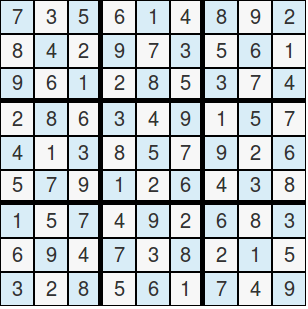You are here:--The #Game of #Maths

# The #Game of #Maths

Try imagining going to a place where there is no electricity. Therefore, we can’t use any electronics for our entertainment. Don’t despair because we can create own amusement with the game of maths.

Sudoku

This game has an interesting history. The origin of this game is from Switzerland. Leonhard Euler invented this game with the name “Latin Squares.” It arrived in Japan where it became popular and got its popular name, which is su (number) and doku (single). Draw a 9×9 grid. Fill in the squares with the numbers 1 to 9 so that the columns, the rows, and the 3×3 boxes will contain the numbers from 1 to 9.Palindrome

A palindrome is a game that a group of people can play. The players don’t need to know much about numbers to play this game because words can be used to play this game. A palindrome is a word or phrase that reads the same backward as forward. An example of a palindrome is the word madam or race car.

The principle of the palindrome of the numbers is the same as the word. We only use numbers instead of letters for this game. 11, 232, 45654, and 9009 are examples of number palindrome.

All numbers have their own palindrome. Let us take 10 for example. Ten is not a palindrome because the number 10 is not equal to 01. However, we can get the palindrome for 10. We add 10 with 01 that equals to 11, and 11 is a palindrome. Let us get the palindrome of 57. 57 + 75 = 132. We will add again the reverse, which is 231, to 132 and that totals to 363. The number 363 is the palindrome of 57. This game is a good way to exercise the brain with addition.Guess the Number

This game is more like a trick. The game is played well with only one person who knows how the game works and will let the others guess the correct answer.

The host of the game will use his or her hands to show the number of fingers to the players. The first answer is always 0 even though the host is showing 3 fingers. The second answer is 3 even though there are 7 fingers being shown. The third answer is 7 no matter how many fingers the host is showing. The rule of the game is to remember the previous number of fingers and that is the answer to the current number. Zero is the first answer because there was no finger shown before the game started.Word Problems

This type of game is done by asking questions that are related to mathematics. The questions should be easy enough to answer but should be asked in a tricky way to make it challenging. The questions should be related to the activity being done to make it more interesting. For example, while traveling, we can ask the question, “If the car is running 100 km/hr, how many hours are we going to travel when there are 100 kilometers left?”We can still have fun even without the use of gadgets by playing these simple games of mathematics.

By |2018-09-06T09:48:36+01:00September 6th, 2018|Categories: Mathematics||0 Comments

## About the Author: Ryan James